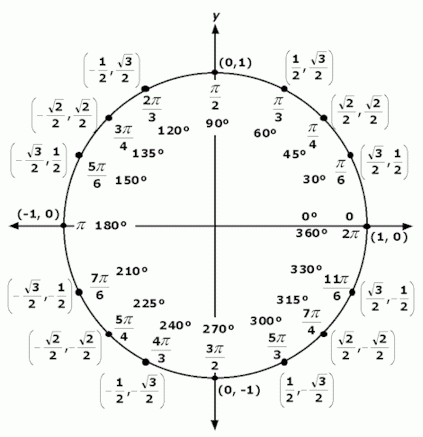GBIC >> PowerBASIC >> Tutorials >> Trigonometry

PowerBASIC Information Center Tutorials
These tutorials were written to help you get a quick, but thorough, understanding of PowerBASIC - the scope of the language as well as it's specific capabilities.

Introduction       Projects       Language           Messages       Functions           Advanced
• Overview
• Examples
• IDE
• Compilation
• Distribution
• Project Files
• DDT Windows
• Controls
• Dialogs
• Help Files
• Resources
• Templates
• Project Shell
• Syntax
• Operators
• Data Types
• Variables
• Scope
• Declarations
• Procedures
• Flow Control
• Windows
• Messages
• Callbacks
• Mouse
• Keyboard
• Dialogs
• Controls
• Subclassing
• Arithmetic
• Trig
• Strings
• Arrays
• Date/Time
• Printing
• Files
• Folders
• Keyboard
• Mouse
• Sound
• System
• Error Traps
• Debugging
• Objects
• Graphics
• Databases
• API
• DLLs
• ASM
• Trigonometric Functions Summary
PowerBASIC provides only four trigonometric functions, as listed in the following table. Note that all of these functions use angles specified as radians, not degrees.

 Angle Argument (ratio out) ` sin, cos, tan ` Ratio Argument (angle out) ` atn `

Right Triangles
All of the trigonometric functions are related to right triangles - those with one 90 degree angle. The longest side (opposite the 90 degree angle) is called the hypotenuse. The other two sides have no standard nomenclature.

The trigonometric functions are simply the 3 possible ratios of all three sides. For any one of the two non-90 degree angles, the terminology of "side adjacent" refers to the non-hypotenuse side, as does the "side opposite".

The three possible ratios, when referring to a specific angle in the triangle, are named sine (opposite/hypotenuse), cosine (adjacent/hypotenuse) and tangent (opposite/adjacent).

Expanded Functions
Fortunately, these values can be used to generate other commonly used trignometric functions, as given in the following equations:

```    csc = cosecant  =  1/sin  =  hypotenuse/opposite
sec = secant    =  1/cos  =  hypotenuse/adjacent
cot = cotangent =  1/tan  =  adjacent/opposite
```

The trignometric functions use radians - not degrees! Conversion factors are:

```    1 degree = 0.0174533 radians
```

Also, here's a diagram showing the relationship of radians and degrees over a complete circle.Trigonometric Functions Listing
Here are one-line descriptions of all the trigonometric functions.

• atn - arctangent (angle whose tangent is a specified number value)
• cos - cosine (side adjacent over hypotenuse)
• sin - sine (side opposite over hypotenuse)
• tan - tangent (side opposite over side adjacent)
Trigonometric Functions Examples
Here are examples for each of the trigonometric functions. The functions are listed in alphabetical order.

• atn - arctangent
```    result = atn(1)           ' result = .7894
```
• cos - cosine
```    result = cos(0.7854)      ' result = 0.707
```
• sin - sine
```    result = sin(0.7854)      ' result = 0.707
```
• tan - tangent
```    result = tan(0.7854)      ' result = 1.0
```

If you have any suggestions or corrections, please let me know.